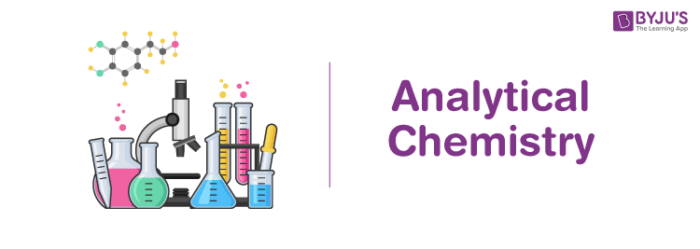# Analytical Chemistry

## What is Analytical Chemistry?

Analytical chemistry is the branch of chemistry that deals with the analysis of different substances.

It involves the separation, identification, and the quantification of matter. It involves the use of classical methods along with modern methods involving the use of scientific instruments.

Analytical chemistry involves the following methods:

• The process of separation isolates the required chemical species which is to be analysed from a mixture.
• The identification of the analyte substance is achieved via the method of qualitative analysis.
• The concentration of the analyte in a given mixture can be determined with the method of quantitative analysis.

Today, the field of analytical chemistry generally involves the use of modern, sophisticated instruments. However, the principles upon which these instruments are built can be traced to more traditional techniques.

## Recommended Videos## Methods Used in Analytical Chemistry

The methods used to determine the identity and the quantity of the analytes in the field of analytical chemistry can be broadly divided into classical and instrumental methods.

### 1. Classical Methods

• There exist many classical methods of checking for the presence or absence of a particular compound in a given analyte. One such example is the acid test for gold.
• Another example of a classical method for qualitative analysis is the Kastle-Meyer test which employs phenolphthalein as an indicator to check for the presence of haemoglobin in the given analyte.
• Flame tests can be used to check for the presence of specific elements in an analyte by exposing it to a flame and observing the change in the colour of the flame.
• Gravimetric analysis is a classical method of quantitative analysis, which can be used in analytical chemistry to determine the amount of water in a hydrate by heating it and calculating the weight of the water lost.
• One of the better known classical methods of quantitative analysis is volumetric analysis (also known as titration). In the titration method, a reactant is added to the analyte till an equivalence point is obtained.

### 2. Instrumental Methods

• Spectroscopy involves the measurement of the interaction between electromagnetic radiation and the atoms or molecules belonging to a sample.
• With the help of electric fields and magnetic fields, the method of mass spectroscopy is used to measure the ratio of the mass of the molecule to its charge.
• A common instrumental method used in the field of analytical chemistry is electrochemical analysis. In this method, the analyte is placed in an electrochemical cell and the voltage or the current flowing through it is measured.
• The interaction between the analyte and energy in the form of heat is studied in the discipline of analytical chemistry known as calorimetry. A calorimeter is an instrument that is used to measure the heat of a chemical reaction.

It can be noted that even biological measurements are made with the help of this branch of chemistry, and this field is known as bio analytical chemistry.

## Branches of Analytical Chemistry

Two sub-branches come under analytical chemistry namely quantitative analysis and qualitative analysis which can be explained as follows. These two methods form the backbone of many educational labs of analytical chemistry.

### 1. Quantitative Analysis

Quantitative Analysis is a method of determining the absolute or relative quantity regarding the concentration of one or more substances present in a sample or compound.

For example, take a sample of an unknown solid substance. The chemists first use “qualitative” methods to identify what type of compound is present in the sample; then he adopts the quantitative analysis procedure to determine the exact amount or the quantity of the compound present in the sample.

Some Quantitative analysis techniques include Gravimetric Analysis and  Volumetric analysis.

### 2. Qualitative Analysis

Quality means the standard or the feature of one substance. Hence, Qualitative analysis method deals with the determination of the quality of a particular compound, irrespective of its quantity or concentration. In simpler words, the qualitative analysis does not measure the amount of the substance but measures the quality of that material. One of the best examples of this type of method is the observation of a chemical reaction, whether there will be a change in colour or not.

The qualitative analysis method can be measured in different ways such as Chemical tests, flame tests, etc. Several such tests are widely used in salt analysis (identification of the cation & anion of inorganic salts).

### 3. Importance of Analytical Chemistry

Analytical chemistry is the branch which is taught in almost all schools and colleges. But the applications of it are made in pharmaceutical industries, food factories, chemical industries, agricultural industries and in scientific laboratories. The tools used for this purpose are quite expensive which one cannot afford at home.

## Applications of Analytical Chemistry

Some important applications of this branch of chemistry are listed below.

• The shelf lives of many medicines are determined with the help of analytical chemistry.
• It is used to check for the presence of adulterants in drugs.
• Soil can be tested to check for appropriate concentrations of minerals and nutrients that are necessary for plant growth.
• It is employed in the process of chromatography where the blood samples of a person are classified.• The concentration of the pesticide residues and the contaminants in a given food sample can also be determined via analytical chemistry.
• It also has many important applications in medicine, with its use in the testing of cholesterol and glucose levels in a blood sample.
• Analytical chemistry is an integral part of forensic science, clinical analysis, and even environmental analysis.